﻿ Extension: precision - FHIR v4.6.0

# Extension: precision

 FHIR Infrastructure Work Group Maturity Level: 1 Informative Use Context: Any

URL for this extension:

`http://hl7.org/fhir/StructureDefinition/quantity-precision`

Status: draft. Extension maintained by: Health Level Seven, Inc. - FHIR Core WG

Explicit precision of the number. This is the number of significant decimal places after the decimal point, irrespective of how many are actually present in the explicitly represented decimal.

Comment:

The presence of this extension does not change conformance expectations with regard to rendering and calculations that use the number - these are still based on the raw decimal value. Applications that perform calculations SHALL ensure that this extension is removed or updated to the correct precision value if the number is changed. Applications should consider using the exponential form (e.g. 2.10e3) on the raw decimal to manage significant figures, but should be mindful of human display when doing so.

Context of Use: Use on Element ID decimal

## Extension Content

Summary

NameFlagsCard.TypeDescription & Constraints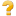precision0..1integerURL = http://hl7.org/fhir/StructureDefinition/quantity-precision
precision: Explicit precision of the number. This is the number of significant decimal places after the decimal point, irrespective of how many are actually present in the explicitly represented decimal.

Use on Element ID decimalDocumentation for this format

Full Structure

NameFlagsCard.TypeDescription & Constraintsextension0..1ExtensionURL = http://hl7.org/fhir/StructureDefinition/quantity-precision
precision: Explicit precision of the number. This is the number of significant decimal places after the decimal point, irrespective of how many are actually present in the explicitly represented decimal.

Use on Element ID decimal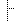extension 0..0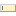url 1..1uri"http://hl7.org/fhir/StructureDefinition/quantity-precision"value[x] 1..1integerValue of extensionDocumentation for this format

XML Template

```<!-- precision -->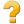<extension xmlns="http://hl7.org/fhir"
url="http://hl7.org/fhir/StructureDefinition/quantity-precision" >
<!-- from Element: extension -->
<valueInteger value="[integer]"/><!--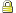1..1 Value of extension -->
</extension>
```

JSON Template

```{ // precision
// from Element: extension
"extension" : [ //  sliced by value:url  in the specified order, Open ]
"url" : "http://hl7.org/fhir/StructureDefinition/quantity-precision", // R!
"valueInteger" : <integer> // C? R! Value of extension
}
```

Summary

NameFlagsCard.TypeDescription & Constraintsprecision0..1integerURL = http://hl7.org/fhir/StructureDefinition/quantity-precision
precision: Explicit precision of the number. This is the number of significant decimal places after the decimal point, irrespective of how many are actually present in the explicitly represented decimal.

Use on Element ID decimalDocumentation for this format

Full Structure

NameFlagsCard.TypeDescription & Constraintsextension0..1ExtensionURL = http://hl7.org/fhir/StructureDefinition/quantity-precision
precision: Explicit precision of the number. This is the number of significant decimal places after the decimal point, irrespective of how many are actually present in the explicitly represented decimal.

Use on Element ID decimalextension 0..0url 1..1uri"http://hl7.org/fhir/StructureDefinition/quantity-precision"value[x] 1..1integerValue of extensionDocumentation for this format

XML Template

```<!-- precision --><extension xmlns="http://hl7.org/fhir"
url="http://hl7.org/fhir/StructureDefinition/quantity-precision" >
<!-- from Element: extension -->
<valueInteger value="[integer]"/><!--1..1 Value of extension -->
</extension>
```

JSON Template

```{ // precision
// from Element: extension
"extension" : [ //  sliced by value:url  in the specified order, Open ]
"url" : "http://hl7.org/fhir/StructureDefinition/quantity-precision", // R!
"valueInteger" : <integer> // C? R! Value of extension
}
```

Constraints

• ele-1: All FHIR elements must have a @value or children (xpath: @value|f:*|h:div)
• ext-1: Must have either extensions or value[x], not both (xpath: exists(f:extension)!=exists(f:*[starts-with(local-name(.), 'value')]))
• ele-1: On Extension.extension: All FHIR elements must have a @value or children (xpath on Extension.extension: @value|f:*|h:div)
• ext-1: On Extension.extension: Must have either extensions or value[x], not both (xpath on Extension.extension: exists(f:extension)!=exists(f:*[starts-with(local-name(.), "value")]))
• ele-1: On Extension.value[x]: All FHIR elements must have a @value or children (xpath on Extension.value[x]: @value|f:*|h:div)